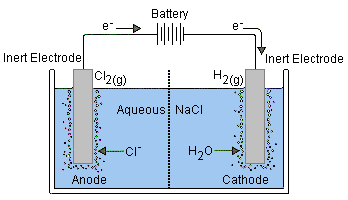# Electrochemical Cells

There are two types of electrochemical cells

• Galvanic, or Voltaic
• Electrolytic

Galvanic cells derives energy from spontaneous redox (oxidation-reduction) reactions.

Electrolytic cells involve non-spontaneous reactions, and thus require an external electron source (for example, a D.C. battery).

Both Galvanic and electrolytic cells will consist of two electrodes (anode, and cathode), and an electrolyte in which the two electrodes are immersed.

## Galvanic Cells

Galvanic cells are traditionally used as sources of DC power.

• Oxidation occurs at the Anode
• Reduction occurs at the Cathode

The reaction at the anode is a source of electrons. The anode is the negative terminal for the galvanic cell.

Remember: Red Cat, An Ox – (reduction cathode, anode oxidation)

### Example of Galvanic Cell

Below is an example of a classic AA battery.

1. MnO2 (s) + H20 + e → MnOOH s + OH (aq)      E° = +0.3832V

2. Zn(OH)2 (s) + 2e → Zn (s) + 2OH (aq)       E° = -1.221VImage by Ohiostandard at English Wikipedia – Transferred from en.wikipedia to Commons by Burpelson AFB using CommonsHelper., CC BY-SA 3.0, https://commons.wikimedia.org/w/index.php?curid=11236033

Galvanic cells have a positive EMF.

EMF = +0.382 – (-1.221)

∴ = 1.6 *(approx 1.5V – standard AA battery)*

## Calculating the Gibbs Free Energy from EMF

Formula: ΔG° = -nFE°

• n = number of moles of electrons transferred
• E° = the standard state of EMF
• F = Faraday’s constant (96,4585 C/mol e), approximately equal to 105 C/mol e

From the previous equations and results:

ΔG° = -nFE°

ΔG° = -(2 mol e)(105 C/mol e)(1.5V)

ΔG° = -300,000J, or -300kJ

The negative value for ΔG° indicates the spontaneity of the reaction.

The larger the value for ΔG°, the further the reaction has to shift from it’s standard conditions to reach equilibrium.

## Calculating the Equilibrium Constant from EMF

This gives an indication of how favorable a reaction is.

Formula: ΔG° = -RTlnKeq

• R = gas constant (8.31 J/mol-k)
• T = standard temperature (298K)
• ln = converted to log = 2.303 (not expected to know this value)

ΔG° = -RT lnx logK

ΔG° = -(8.31)(298)(2.303)(log K)

ΔG° = -2500(2.3)(log K)

ΔG° = -6000(log K)

From before:

-300,000 = -6000(log K)

50 = log K

By raising both sides as exponents with base 10:

10log K = K = 1050

## Electrolytic Cells

This is an electrochemical cell that uses external energy (external power source) to drive a normally non-spontaneous reaction. That is, to apply a reverse voltage to a voltaic cell.

In comparison to galvanic cells, the electrodes of an electrolytic cell can be placed in a single compartment containing the molten of aqueous electrolyte.

Since the external battery is what drives the electrons through the circuit, the electrodes will match the positive and negative terminal of the battery. While the anode remains the site of oxidation, it becomes the positive terminal, and the cathode becomes the negative terminal.Electroplating always occurs at the cathode.

We can calculate the amount of metal plated on the cathode from the charge or current that passes through the cell, and in fact, they are proportional in the following relationship:

Q (charge of e from battery) = Q (charge of e from reduction)

Formula: It = nF

• I is the current expressed in amperes or coulombs/second
• n is the moles of electrons
• F is Faraday’s constant (approx 105)

Question: How much platinum metal in grams is deposited when 5 amps of current is passed through an electrolyte for 1 hour?

It = nf

5(3600) = n(105)

18,000 = n(100000)

n = 0.18 mol e

(0.18 mol e)(1 mol Pt / 2 mol e)* = 0.09 mol Pt

(0.09 mol Pt)(195 g Pt/mol Pt) = Approx. 18g Pt

* Platinum forms a 2+ ion, so there are two moles of e consumed. Divide by 2 to get moles of platinum produced.

## Electrode Potential

Ecell = oxidation potential + reduction potential

1. The electrode potential cannot be determined in isolation

2. The electrode potential depends on the concentrations of the substances, the temperature, and the pressure in the case of the gas electrodes

# Summary of Electrochemical Cells

Electrolytic
(Nickel-iron cell)
Galvanic/Voltaic
(Zinc-cerium cell)
Oxidation
Occurs at Anode Anode
Sign of Terminal Positive (+) Negative (-)
EMF E° = -0.52 V E° = +0.76 V
Reduction
Occurs at Cathode Cathode
Sign of Terminal Negative (-) Positive (+)
EMF E° = -0.89 V E° = +1.44 V
Electrolytic Cell
EMF Signage Negative (-) Positive (+)
EMF Value E° = -1.41 V E° = +2.20 V
Electron Flow From anode to cathode From anode to cathode
Gibbs Free Energy
(ΔG°)
Positive (+) Negative (-)
Spontaneous No Yes